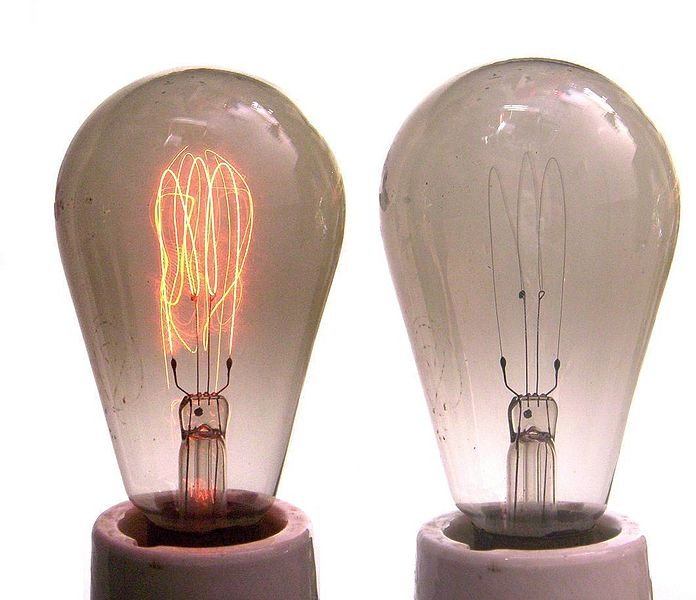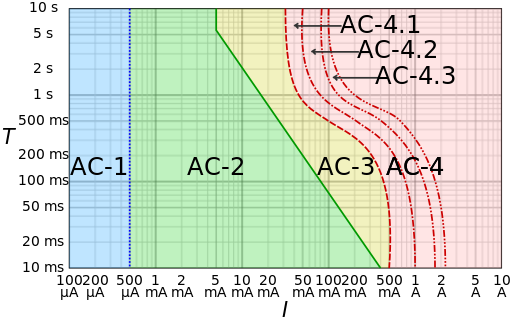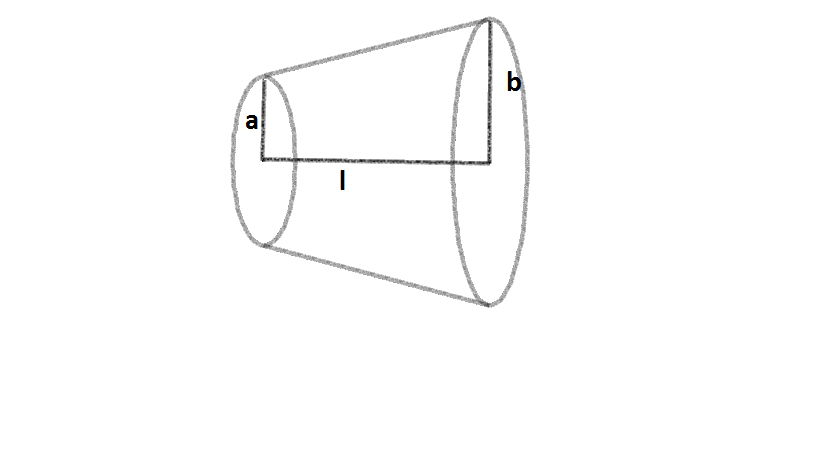Electricity and Magnetism

# Resistors: Level 1-3 ChallengesAn incandescent light bulb gets hot because

###### Image credit: Wikipedia UlfbastelAC-1: imperceptible
AC-2: pain, but no muscle contraction
AC-3: pain with muscle contraction
AC-4: possible irreversible effects
AC-4.1: up to 5% probability of ventricular fibrillation
AC-4.2: 5-50% probability of fibrillation
AC-4.3: over 50% probability of fibrillation

The table above shows the corresponding physiologic effects when a person is electrocuted by an alternating current according to the time duration of the shock and the magnitude of current that flows through his/her body. The resistance of a human body (when the skin is dry) when shocked by 110 Volts is known to be about 2,000 Ohms. What will happen when Bryan gets electrocuted for 0.5 seconds while plugging his Xbox into a 110-Volt outlet?

Details and Assumptions

• Ventricular fibrillation is a type of heart attack.
###### The table was copied from Wikipedia.A metallic cylindrical conductor is used to produce some heat by applying a constant voltage between it's two ends. It's cold, and you need to double the heat released. Which of the following is the most appropriate thing to be done?Inside the electric eel there is a special organ that contains many EMF generating cells. These cells are called electroplaques. It is discovered that a typical eel contains $140$ rows of $5000$ electroplaques, where each electroplaque is capable of generating an EMF of $0.15$ volts. The internal resistance of an electroplaque is approximately $0.25\ \Omega$. If the water surrounding the eel has a resistance of $800\ \Omega$, how strong is the maximum current (in Amperes) produced by the electric eel in the water?

This is my favourite circuits problem from Fundamentals of Physics by Halliday, Resnick, Walker.

Clarification: There are $140$ rows with $5000$ electroplaques, and each row is connected in parallel.A conductor of length $l$ has a uniform cross section.

The radius of cross section varies linearly from $a$ to $b$.The resistivity of the material is $\rho$.

Find the resistance of the conductor across its ends in Ohms.

Details and Assumptions

• $\rho$=$6.28\times$$10^{-8}$$\Omega$m
• $l$ (length of conductor) =10 cm
• $a$=2 cm and $b$=5 cm
×